# [Solved] AttributeError: module ‘keras.preprocessing.image‘ has no attribute ‘load_img‘

Original code:

``````from keras.preprocessing import image
x           =   image.img_to_array(img)``````

``AttributeError: module 'keras.preprocessing.image' has no attribute 'load_img'``

Reason: The keras version has been updated.

Solution:

``````from keras.utils import image_utils
x           =   image_utils.img_to_array(img)
``````

# [Solved] AttributeError: module ‘tensorboard.summary._tf.summary‘ has no attribute ‘merge‘

The environment is TensorFlow2.9

The code to be run on github The TensorFlow environment is 1.x

Many apis cannot be called

Original code

``````train_summary_op = tf.summary.merge([loss_summary])
``````

Error reporting in method 1

``````train_summary_op = tf.compat.v1.summary.merge([loss_summary])
``````

display

``````TypeError: Tensors in list passed to 'inputs' of 'MergeSummary' Op have types [bool] that do not match expected type string.
``````

If it can’t be solved, I will try

``````import tensorflow as tf
``````

Modified as

``````import tensorflow._api.v2.compat.v1 as tf
tf.disable_v2_behavior()
``````

Finally, solve the problem successfully

# [Solved] Operator Not Allowed In Graph Error & Attribute Error Tensor object has no attribute numpy

The reason for the above error when compiling custom functions is that tf2.x’s keras.compile does not support specific values by default

Questions

When using the wrapping method to customize the loss function of the keras model and need to calculate accuracy metrics such as precision or recall, or need to extract the specific values of the inputs y_true and y_prd (operations such as y_true.numpy()), an error message appears:

`OperatorNotAllowedInGraphError: using a `tf.Tensor` as a Python `bool` is not allowed: AutoGraph did convert this function. This might indicate you are trying to use an unsupported feature.`

Or

` AttributeError: 'Tensor' object has no attribute 'numpy'`

Solution:

Pass in parameters in the compile function:

run_eagerly=True

Reason:

Tf2.x enables eager mode by default, namely eager execution, that is, dynamic calculation graph. Compared with the static calculation graph of tf1.x, the advantage of eager mode is that it is convenient for debugging, which can easily print tensor values ​​and evaluate results; and Numpy interacts well, and the conversion between tensor and ndarray is convenient and even universal. The tradeoff is that it runs significantly slower. After the static calculation graph is defined, it is almost always executed with C++ code on the tensorflow core, so the calculation efficiency is higher and the speed is faster.

Even so, run_eagerly defaults to False in the model.compile method, which means that the logic of the model is encapsulated in tf.function, which achieves faster computational efficiency (the autograph mechanism converts the dynamic computational graph through the @tf.function wrapper). is a static computation graph). But the @tf.function wrapper requires the function to use basic tf operations, not other operations in python or even functions from other packages, so the first error occurs when calling functions such as sklearn.metrics’ accuracy_score or imblearn.metrcis’ geometric_mean_score function. The second error occurs when using the y_true.numpy() method. The fundamental reason is that the model.compile method does not support the above operations after the static calculation graph converted by the @tf.function wrapper, although tf2.x enables the use of dynamic calculation graphs by default.

After passing run_eagerly=True to the model.compile method, the dynamic calculation graph is used to run, and the above operations can be performed normally. The disadvantage is that the dynamic calculation graph has the disadvantage of low operation efficiency.

# tensorflow2.3 InvalidArgumentError: jpeg::Uncompress failed [How to Solve]

```tensorflow2.3 InvalidArgumentError: jpeg::Uncompress failed
[[{{node decode_image/DecodeImage}}]] [Op:IteratorGetNext]```

Solution:
check whether the picture is damaged before training:

``````import tensorflow as tf
from tensorflow import keras
from tensorflow.keras import layers
import os

num_skipped = 0
for folder_name in ("Fruit apples", "Fruit bananas", "Fruit oranges"):
folder_path = os.path.join(".\data\image_data", folder_name)
for fname in os.listdir(folder_path):

fpath = os.path.join(folder_path, fname)

try:
fobj = open(fpath, mode="rb")
is_jfif = tf.compat.as_bytes("JFIF") in fobj.peek(10)

finally:
fobj.close()

if not is_jfif:
num_skipped += 1
# Delete corrupted image
os.remove(fpath)

print("Deleted %d images" % num_skipped)
``````

Delete the damaged picture and train again to solve the problem
if an error is prompted again, use:

``````# Determine if an image is corrupt from local
def is_valid_image(path):
'''
Check if the file is corrupt
'''
try:
bValid = True
fileObj = open(path, 'rb')  # Open in binary form
bValid = False
elif buf[6:10] in (b'JFIF', b'Exif'): # ASCII code of "JFIF"
if not buf.rstrip(b'\0\r\n').endswith(b'\xff\xd9'): # whether it ends with \xff\xd9
bValid = False
else:
try:
Image.open(fileObj).verify()
except Exception as e:
bValid = False
print(e)
except Exception as e:
return False
return bValid

num_skipped = 0
for folder_name in ("fruit-apple", "fruit-banana", "fruit-orange"):
#os.path.join() joins two or more pathname components
folder_path = os.path.join(". \data\image_data", folder_name)
# os.listdir(path) lists the subdirectories under this directory
for fname in os.listdir(folder_path):
fpath = os.path.join(folder_path, fname)
flag1 = is_valid_image(fpath)
if not flag1:
print(flag1)
print(fpath)#Print the path and name of the error file

``````

Adjust the error file and train again to solve the problem.

# [Solved] RuntimeError: Cannot clone object ＜keras.wrappers.scikit_learn.KerasClassifier object…

Today, I debugged the code and found this error. The complete contents are as follows:

RuntimeError: Cannot clone object < keras. wrappers. scikit_ learn. KerasClassifier object at 0x000003A783733F33>, as the constructor either does not set or modifies parameter layers

No error is reported when the code is found. It is normal

The code is then investigated:

``from keras.wrappers.scikit_learn import KerasRegressor``

Change to:

``from tensorflow.keras.wrappers.scikit_learn import KerasRegressor``

Problem solved!

# [Solved] Error occurred when finalizing GeneratorDataset iterator: Failed precondition: Python interpreter st

When using tensorflow.keras, this error is often reported during model training:

``````tensorflow/core/kernels/data/generator_dataset_op.cc:107] Error occurred when finalizing GeneratorDataset iterator: Failed precondition: Python interpreter state is not initialized. The process may be terminated.
[[{{node PyFunc}}]]
``````
```tensorflow/core/kernels/data/generator_dataset_op.cc:107] Error occurred when finalizing GeneratorDataset iterator: Failed precondition: Python interpreter state is not initialized. The process may be terminated.

[[{{node PyFunc}}]]```

According to my own experience, there are several reasons for this errory:

1. The input image_size and input_shape does not match or is not defined when the model is built. Note that the input_shape must be defined when defining the first layer of the convolutional layer, e.g.

``````    model = keras.models.Sequential([
# Input image [None,224,224,3]
# Convolution layer 1: 32 5*5*3 filters, step size set to 1, fill set to same
# Output [None,32,32,3]
activation='relu', input_shape=(224, 224, 3)),``````

2. There is also train_generator and validate_generator related parameters must be consistent, such as batch_size, target_size, class_mode, etc.

3. The configuration limit itself, try to change the batch_size to a smaller size, or even to 1

4. The last program did not finish completely, finish all python programs to see.

# TensorFlow-gpu Error: failed call to cuInit: CUDA_ERROR_NO_DEVICE: no CUDA-capable device is detected

Error Messages:

`failed call to cuInit: CUDA_ERROR_NO_Device: no CUDA capable device is detected `
this is also what I encountered when running a CNN SVM classifier program of tensorflow GPU today. It’s not the problem of the program. It’s our graphics card.

Solution:

``````import tensorflow as tf

config = tf.compat.v1.ConfigProto()
config.gpu_options.allow_growth = True
session = tf.compat.v1.InteractiveSession(config=config)
``````

Just add these lines of code to the head of the code, and you don’t need to write this code below`os.environ['CUDA_VISIBLE_DEVICES'] = '/gpu:0'`

# [Solved] original_keras_version = f.attrs[‘keras_version‘].decode(‘utf8‘)

windows system:
original_keras_version = f.attrs[‘keras_version’].decode(‘utf8’)
1. error:

``````load_weights_from_hdf5_group
original_keras_version = f.attrs['keras_version'].decode('utf8')

AttributeError: 'str' object has no attribute 'decode'
``````

2. Cause analysis

When installing tensorflow, the default installed h5py is 3.1.0, and an error is reported because the TF you installed does not support an excessively high version of h5py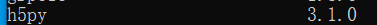3. Solutions

1. Uninstall h5py3 Version 1.0, installing h5py2.0 Version 10.0.2. Restart the compiler

``````pip install h5py==2.10.0
``````

**

**
cause of the problem: check whether we use dataparallel for multi GPU during training. The model generated by this method will automatically add key: module
observe the error message:you can find that the key values in the model are more modules

Solution:
1. Delete the module

``````    gentmps=torch.load("./saved_models/generator_%d.pth" % opt.epoch)
from collections import OrderedDict
new_gens = OrderedDict()
new_diss = OrderedDict()
for k, v in gentmps.items():
name = k.replace('module.','') # remove 'module.'
new_gens[name] = v #The value corresponding to the key value of the new dictionary is a one-to-one value.
for k, v in distmps.items():
name = k.replace('module.','') # remove 'module.'
new_diss[name] = v #The value corresponding to the key value of the new dictionary is a one-to-one value.
``````

# [Solved] Jupyter notebook use pyLDAvis Error: modulenotfounderror: no module named ‘pyLDAvis’‘

Background of the problem

Getting started with Python
try to use pyldavis for simple topic extraction;

Problems and related codes

Pyldavis has been installed, as shown in the figure (Annotated):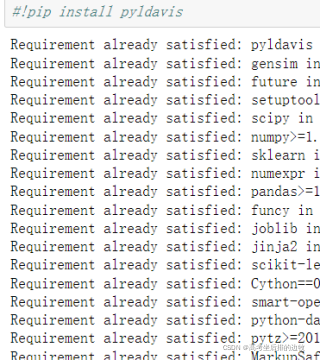but an error occurs when entering the following statement:
error code segment:

``````import pyLDAvis
import pyLDAvis.sklearn
pyLDAvis.enable_notebook()
pyLDAvis.sklearn.prepare(lda,tf,tf_vectorizer)
``````

Screenshot of specific error reporting: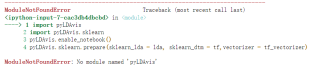Solution:

Some bloggers suggested that the installation was not successful. I installed it as an administrator. I operated it, but it was useless
in fact, the final solution is very simple. I found that the kernel used by my Jupiter notebook is wrong. I used a virtual environment I set up before. Just change it to the default environment (my one is named python3).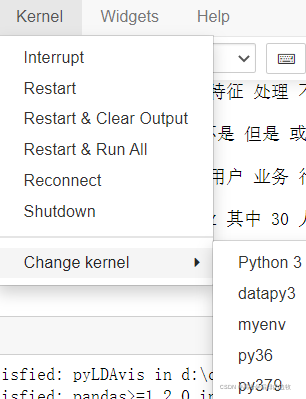# How to Solve Keras calls plot_model error

1. Error information

When building the neural network model, you can call plot in keras_ The model module draws a schematic diagram of the model to facilitate the adjustment of the model structure:

``````from tensorflow.keras.models import Model
from tensorflow.keras.utils import plot_model
model = Model(dense_inputs+sparse_inputs, output_layer)
plot_model(model, "fm_model.png", show_shapes=True)
``````

As a result, the following error messages appear:

(‘Failed to import pydot. You must pip install pydot and install graphviz (ht

Understand the error message: the installation is complete without pydot and graphviz packages

2. Solutions

2.1 installation of graphviz package

``````pip install graphviz
``````

In Windows Environment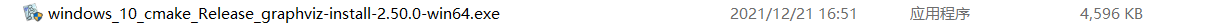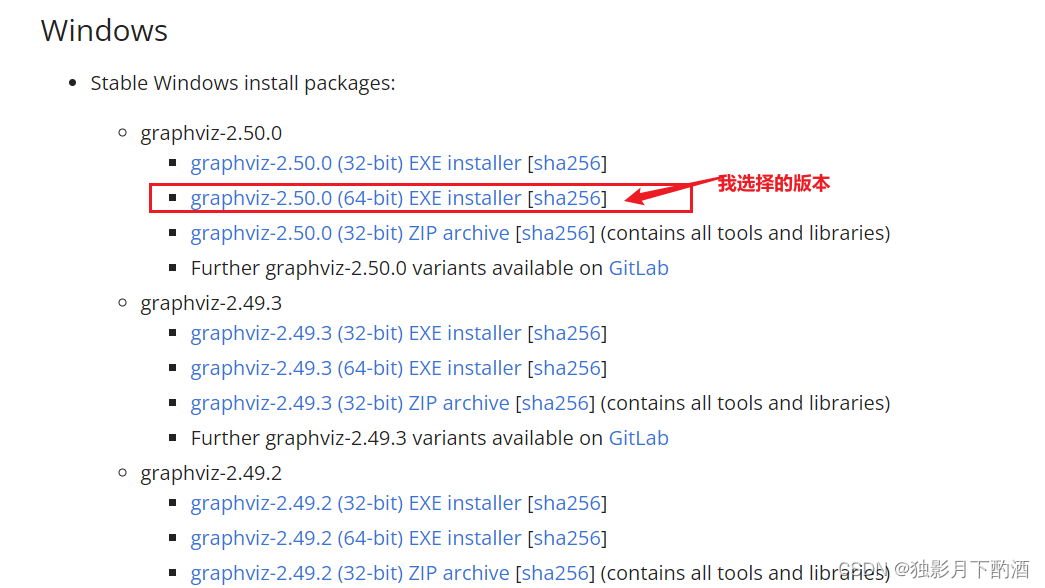2.3 configuring environment variables for graphviz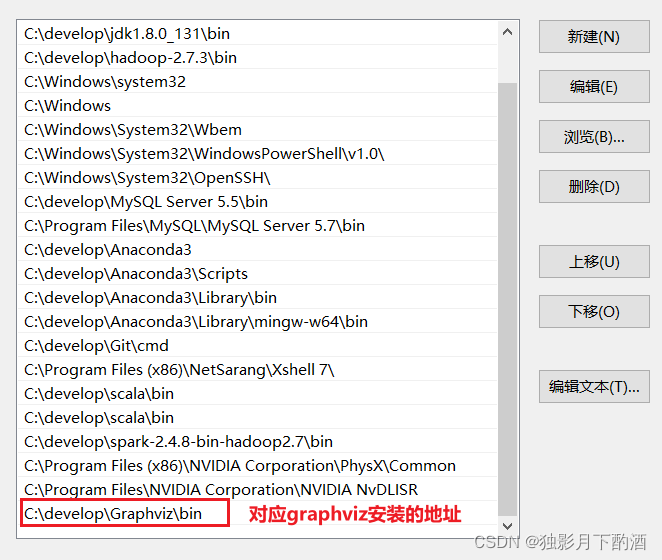2.4 installing pydot package

``````pip install pydot-ng
``````

2.5 restart development tools

Restart the IDE or other development tools (Jupiter notebook) with immediate effect.

3. Summary

1. Installing pydot and graphviz packages directly according to the error message does not work

2. You need to go to the website to download the corresponding EXE file or zip file. After installation, specify the environment variables

3. Don’t forget to restart your ide or other development tools

# [Solved] Python2 Install tensorflow Error: class DescriptorBase(metaclass=DescriptorMetaclass), SyntaxError: invalid syntax

When Python 2 installs tensorflow, test after the installation is completed:

``````import tensorflow as tf
``````

Will report an error:

Traceback (most recent call last):
File “<stdin>”, line 1, in <module>
File “/home/zhaokai/.local/lib/python2.7/site-packages/tensorflow/__init__.py”, line 28, in <module>
from tensorflow.python import pywrap_tensorflow  # pylint: disable=unused-import
File “/home/zhaokai/.local/lib/python2.7/site-packages/tensorflow/python/__init__.py”, line 52, in <module>
from tensorflow.core.framework.graph_pb2 import *
File “/home/zhaokai/.local/lib/python2.7/site-packages/tensorflow/core/framework/graph_pb2.py”, line 7, in <module>
from google.protobuf import descriptor as _descriptor
``pip install protobuf==3.17.3``